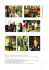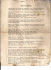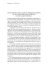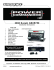# Improved Deadbeat Current Controller with a Repetitive

## Transcription

Improved Deadbeat Current Controller with a Repetitive
```64
Journal of Power Electronics, Vol. 11, No. 1, January 2011
JPE 11-1-10
Improved Deadbeat Current Controller with a
Repetitive-Control-Based Observer for PWM
Rectifiers
Jilei Gao† , Trillion Q. Zheng∗ , and Fei Lin∗
†∗ School
of Electrical Engineering, Beijing Jiaotong University, Beijing, China
Abstract
The stability of PWM rectifiers with a deadbeat current controller is seriously influenced by computation time delays and lowpass filters inserted into the current-sampling circuit. Predictive current control is often adopted to solve this problem. However,
grid current predictive precision is affected by many factors such as grid voltage estimated errors, plant model mismatches, dead
time and so on. In addition, the predictive current error aggravates the grid current distortion. To improve the grid current predictive
precision, an improved deadbeat current controller with a repetitive-control-based observer to predict the grid current is proposed
in this paper. The design principle of the proposed observer is given and its stability is discussed. The predictive performance
of the observer is also analyzed in the frequency domain. It is shown that the grid predictive error can be decreased with the
proposed method in the related bode diagrams. Experimental results show that the proposed method can minimize the current
predictive error, improve the current loop robustness and reduce the grid current THD of PWM rectifiers.
Key Words: Deadbeat current control, PWM rectifiers, Repetitive control, Time delay
I. I NTRODUCTION
When compared with conventional diode rectifiers and
phase-controlled rectifiers, PWM rectifiers have the advantages
of low current harmonic distortions, a high input power
factor and the ability to have a bi-directional power flow. For
these reasons, PWM rectifiers have been playing important
roles as active front end rectifiers in the fields of adjustable
speed drives, grid-connected converters for renewable energy
systems, and power conditioning and transmission equipment.
As the grid side converters of main traction systems and
onboard power supply systems, they have been widely used
in modern ac drive electric locomotives –.
Various control strategies for PWM rectifiers have been
proposed to regulate the dc link voltage while improving
the quality of the input ac current. A double closed-loop
control method is often adopted where the outer loop is a dc
voltage loop and the inner loop is a current loop. When using
this method the system performance is largely determined
by the inner current loop. The hysteresis current control
scheme features a fast response, excellent accuracy and easy
implementation. However, the variable switching frequency
imposes excessive difficulty in designing the main circuit of
the converters , . The ramp comparison control using
Manuscript received Sep. 28, 2009; revised Nov. 14, 2010
† Corresponding Author: [email protected]
Tel: +86-010-5168-4051, Fax: +86-010-5168-4029, Beijing Jiaotong Univ.
∗ School of Electrical Eng., Beijing Jiaotong University, China
error between the reference current and the actual current.
The PI parameters tuning are also required to suit the load
conditions . It is widely known that the steady-state ac
error limitation of the ramp comparison control was overcome
by “the synchronous frame current control method” proposed
by Schauder and Caddy and refined by Rowan and Kerkman
, . Proportional-resonant current control can also realize
a zero steady-state ac error by utilizing its characteristic of
infinite gain at the grid frequency but without a rotating
frame transformation. However, the poles assignment should
be made to obtain a high performance and the tuning process
is not straightforward. The performance also largely depends
on the microprocessor capability and the discrete method of
the resonant controller –.
A deadbeat current control algorithm calculates the converter duty cycle according to a plant discrete model in every
sampling interval to make sure the grid current tracks the
reference in the next interval. It can be easily implemented
on digital processors, and it has the excellent characteristic
of a fast current response. However, the inherent sampling
and computation time delay of digital control and a low-pass
filter in the current sample circuit degrades the performance
of the converters and reduces its robustness . An ac
filtering inductor parameter must be obtained exactly in the
controller; otherwise the mismatch will influence the stability.
The sampling point should be set just ahead of the controlling
point by the period of the total delay to reduce its effects
, . However, the delay has to be measured accurately.
Improved Deadbeat Current Controller with a Repetitive-Control-Based Observer for PWM Rectifiers
Fig. 2.
65
Control block diagram of the current-loop.
as , , :
Fig. 1.
Power circuit of single-phase PWM rectifiers.
A predictive current controller is often adopted to compensate
for the time delay , . However, the one-step predictive
current method is essentially an open-loop current observer
and the prediction error is not converged as in . The
current predictive value is influenced by factors such as plant
uncertainty, grid voltage estimated error, dead-time, etc. The
use of a closed loop predictive observer for predictive deadbeat control is discussed in . Also, an adaptive self-tuning
load model was used in a predictive current controller to ensure
converter stability and robustness , .
Recently, a repetitive control (RC), based on the internal
model principle, has been successfully applied in the fields of
UPS, active power filters, inverters and so on –. RC is
an effective method to exactly track periodic reference signals
and to eliminate periodic errors.
In this paper, an improved deadbeat current control method
with a repetitive-control-based observer is proposed, which can
significantly improve the grid current predictive precision in
the presence of uncertainties and disturbances. In section II,
the current stability of conventional deadbeat control for PWM
rectifiers is analyzed considering a one sampling-period delay
and a current sample filter. The shortcomings of the openloop current observer used to compensate the delay are also
analyzed in section II. Further, in section III the new observer
based on repetitive control is proposed and the design method
is given. In section IV, experiments are made to validate
the proposed method and comparisons are given between the
open-loop predictive current method and the proposed method.
The experimental results show that the proposed method is
correct and effective.
II. P ERFORMANCE A NALYSIS OF D EADBEAT C URRENT
C ONTROL
A. Discrete model of PWM Rectifiers
Fig.1 shows the power circuit of a single-phase PWM rectifier. In this figure, S1 –S4 represent the power semiconductor
devices; ug and ur are the grid side and the converter side
voltage respectively; L is the ac side filtering inductor; uL and
iL represent the voltage and the current of the filtering inductor
respectively; Cd is the dc link capacitor and udc is the dc link
output voltage.
Assuming the PWM rectifier switching period is a constant
value Ts , in the switching period [kTs , (k + 1)Ts ], the discrete
model of the PWM rectifier current-loop plant can be written
av
uav
r (k|k + 1) = ug (k|k + 1) −
L
[iL (k + 1) − iL (k)]
Ts
(1)
where, ur av (k|k +1) and ug av (k|k +1) denote the average value
of the converter side voltage and the grid side voltage over
the switching period [kTs , (k + 1)Ts ], respectively. iL (k) and
iL (k + 1) denote the instantaneous value of the grid current at
the sampling points kTs and (k + 1)Ts , respectively.
The aim of a current controller is to make the inductor
current at the sampling point (k + 1)Ts equal to the reference current value iL re f (k + 1) at the end of the switching
period[kTs , (k + 1)Ts ]. Therefore the converter side reference
voltage in this period can be written as shown in (2) according
to the basic principle of the conventional deadbeat current
control strategy .
L re f
f
av
(2)
· [i (k + 1) − iL (k)]
ure
r (k) = ûg (k|k + 1) −
Ts L
where, ûav
g (k|k + 1) denotes the average value of the grid voltage in the period [kTs , (k + 1)Ts ] and it can be estimated from
the previously measured values using linear extrapolation.
B. Stability analysis with the conventional deadbeat current
control method
A delay always exists between the sampling of the inductor
current and the generation of the converter side voltage,
because time for data conversions by the A/D converters and
calculations by the microprocessors is required. For the sake of
simplicity, one sampling-period delay is assumed to analyze
the problem. A discrete control block diagram of a singlephase PWM rectifier is shown in Fig.2.
The delay of one sampling-period is modeled by the z−1
block. The ZOH equivalence transfer function of the plant is
shown in (3) .
G p (s)
Ts
1
]= ·
.
(3)
s
L z−1
The deadbeat controller D(z) of the current-loop can be
written as:
L̂
L
D(z) =
= kL
(4)
Ts
Ts
G p (z) = (1 − z−1 ) · Z[
where, L̂ is the ac filtering inductance used in the controller
and kL = L̂/L is defined to denote the difference between the
inductance used in the controller and the actual value.
A transfer functions of the current-loop without considering
the sampling-period delay and with a one sampling-period
delay can be described by (5) and (6), respectively.
G1 (z) =
z · D(z) · G p (z)
1 + D(z) · G p (z)
(5)
66
Journal of Power Electronics, Vol. 11, No. 1, January 2011
Fig. 4.
Fig. 3.
Timing schematic of deadbeat current controller with open-loop
current observer.
Root locus of current-loop gain with one sampling-period delay in
case of different kT values.
G2 (z) =
D(z) · G p (z)
.
1 + D(z) · z−1 · G p (z)
f
av
ure
r (k) = ûg (k + 1|k + 2) −
(6)
Submitting (3) and (4) into (5) and (6), the stability condition of the current loop obtained is 0 < kL < 2 and 0 < kL < 1
without considering the delay and with a one sampling-period
delay, respectively. It can be seen that the robustness for
inductance mismatch of the current loop is reduced because
of the existence of the delay.
A low-pass filter is often adopted to suppress the influence
of switching noises in a current sampling circuit. The filtering
transfer function H(s) can be written as (7) assuming that the
filtering time coefficient is T f .
H(s) =
1
.
Tf s + 1
(7)
Defining the time coefficient kT = T f /Ts , the discrete transfer function of the plant and the low-pass filter using ZOH
can be written as:
GH(z) =
1
z−1
Ts
).
· (−kT +
+ kT ·
− 1
L
z−1
z − e kT
(8)
The current closed-loop transfer function can be described
by (9).
D(z) · G p (z)
G3 (z) =
.
(9)
1 + D(z) · z−1 · GH(z)
Root locus diagrams of a current-loop with a one samplingperiod delay in the cases of kT = 0.5, 1, 1.5 and 2 respectively
are shown in Fig.3. It is shown that the stability region of kL
is decreased further because of the current sampling filter.
C. Shortcomings of deadbeat control with an open-loop current observer
In order to eliminate the effect of a one sampling-period
delay, a modified deadbeat current control method with an
open-loop current observer, which is called predictive current
control in the literatures was proposed as shown in (10). A
timing schematic of a deadbeat controller with an open-loop
current observer is shown in Fig.4. The sampling point is
set on the instant when the DSP timer counter register value
becomes zero to eliminate the switching noises .
L̂ re f
· [i (k + 2) − îL (k + 1)] (10)
Ts L
where, the grid current îL (k + 1) at (k + 1)Ts instant can be
predicted according to (11):
Ts
re f
· [ûav
(11)
g (k|k + 1) − ur (k − 1)] + iL (k)
L̂
while the actual grid current at (k + 1)Ts instant can be
described as,
Ts
av
iL (k + 1) = · [uav
(12)
g (k|k + 1) − ur (k|k + 1)] + iL (k).
L
By comparing (11) and (12), it can be concluded that
the predictive current value is influenced by the grid voltage
estimated error, the plant model mismatch and the dead-time,
etc. Assuming ∆u(k|k+1) represents the equivalent voltage
error caused by the factors mentioned above, the predictive
current error e(k + 1) at the (k + 1) Ts instant can be written
as:
Ts
e(k + 1) = ∆u(k|k + 1).
(13)
L
The actual grid current value at (k+2)Ts instant can be
described as:
Ts
av
iL (k + 2) = · [uav
g (k + 1|k + 2) − ur (k + 1|k + 2)] + iL (k + 1).
L
(14)
According to (10) and (14), the grid current tracking error
∆i(k + 2) at (k + 2)Ts instant can be expressed as:
îL (k + 1) =
∆i(k + 2) = e(k + 1) + e(k + 2).
(15)
From (15), it is known that the predictive current error
will increasingly distort the grid current and increase the loworder current harmonics proportion. Therefore, improving the
predictive current precision is an effective method to minimize
the grid current harmonics with a predictive current controller.
III. I MPROVED D EADBEAT C URRENT C ONTROL M ETHOD
WITH THE P ROPOSED O BSERVER
A. Proposed repetitive-control-based observer
From the analysis in section II, it is shown that the predictive
current value is seriously affected by the grid voltage extrapolation error, the dead-time and the plant model mismatch.
It is worth emphasizing that all of these disturbances are
periodic signals at the grid voltage frequency. It is well known
67
Improved Deadbeat Current Controller with a Repetitive-Control-Based Observer for PWM Rectifiers
Fig. 5.
Block diagram of repetitive-control-based observer.
that repetitive control based on the internal mode principle
is an effective control method to eliminate periodic external
disturbances. A closed-loop current observer using repetitive
control is proposed in this section to improve the predictive
current precision.
A control diagram of the proposed observer is shown in
Fig.5. The basic repetitive controller can be written as:
R(z) =
1
1
=
1 − Q(z) 1 − kq z−N
(17)
Therefore the compensator C(z) is chosen as kr zN+1 which
has the characteristics of a one sampling-period lead to compensate for the delay of the plant. kr is the loop gain of the grid
current observer. Then, the current observer can be obtained
as shown in (18) according to Fig.5.
ˆ = I(z)G(z) + F(z)G(z) + R(z)C(z)G(z)E(z)
I(z)
(18)
where, I(z) and F(z) are the feed forward compensators which
are the same as the expression shown in (11) with the openloop predictive current method.
ˆ
E(z) = I(z) − I(z).
(19)
Without considering F(z), the closed-loop transfer function
of the observer Go (z) can be written as,
Go (z) =
G(z) + R(z)C(z)G(z)
.
1 + R(z)C(z)G(z)
Fig. 7.
Control block diagram of improved deadbeat controller with
repetitive-control-based observer.
prefect tracking at grid frequency. The current observer can
eliminate the disturbance produced by the factors analyzed in
the former section. A control block diagram of the improved
deadbeat controller with a repetitive-control-based observer is
shown in Fig.7.
B. Comparisons with an open-loop current observer
The predictive current value using the proposed observer at
the (k + 1)Ts instant can be obtained as:
Ts
re f
· [ûav
g (k|k + 1) − ur (k − 1)] + iL (k)
L̂
kr z−N+1
+
[iL (k) − îL (k)].
(22)
1 − kq z−N
îL (k + 1) =
The predictive current error can be written as shown in (23)
by subtracting (22) from (12).
(20)
e(k + 1) =
The observer stability condition is that the roots of the
characteristic polynomial of the closed-loop transfer function,
1 + R(z)C(z)G(z), are inside the unit circle. The stability
condition can be calculated as,
0 < kr < 1.98.
Bode diagram of the proposed observer.
(16)
where, kq is usually a low-pass zero-phase filter or a constant
smaller than 1 to ensure that the observer is stable. It results
in a tradeoff between tracking accuracy and system robustness
. For simplicity of analysis, it is chosen as a constant 0.98
here. N is the sampling times in a grid fundamental period.
The transfer function C(z) in Fig. 5 aims to compensate the
frequency characteristics of the observer plant G(z) which is
the one sampling-period delay shown in (17):
G(z) = z−1 .
Fig. 6.
kr z−N+1
Ts
∆u(k|k + 1) −
e(k).
L
1 − kq z−N
The error transfer function which represents the frequency
characteristics of the predictive current error e(k + 1) versus
the equivalent voltage error ∆u(k|k + 1) with the proposed
observer based on repetitive control is shown in (24).
(21)
However, the condition shown in (21) can just ensure that
the current observer is stable. To guarantee that the whole
current loop is stable, the value of kr must be much smaller
than 1.98 as shown in (21). Here kr is chosen as 0.1. A Bode
diagram of the proposed current observer closed-loop transfer
function is plotted in Fig.6 when kr is equal to 0.1. It presents
(23)
Geclosed (z) =
1 − kq z−N
Ts
·
.
L 1 + (kr − kq )z−N
(24)
The error transfer function with an open-loop observer can
be concluded by (13) as,
Geopen (z) =
Ts
.
L
(25)
68
Fig. 8.
Journal of Power Electronics, Vol. 11, No. 1, January 2011
Bode diagram of the error transfer function with open-loop and the
proposed observer.
(a)
(a)
(b)
Fig. 10. Waveforms of grid voltage and current without current observer
(a) in condition of kT = 1 and kL = 1 (b) in condition of kT = 1 and
kL = 0.95.
(b)
Fig. 9. Waveforms of grid current reference value and actual value (a) with
repetitive current controller combined with deadbeat control (b) with the
improved deadbeat control proposed in this paper.
When the switching frequency is 5 kHz and the ac filtering
inductance is 10.4mH, the frequency characteristics of the
error transfer function of the open-loop and the proposed
observer in this paper are shown in Fig.8 with a dash line and a
continuous line, respectively. It is obviously that the predictive
current error caused by the equivalent voltage error is much
smaller with the proposed observer than with the open-loop
one at the grid voltage integer times frequency. As a result,
the grid current will have lower low-order harmonics with the
proposed method.
C. Simulation Comparisons with the current-loop repetitive
control
Repetitive control has rejection capability against repetitive disturbances with a known period. However, it has
the disadvantage of a slow dynamic response because the
input error of the repetitive controller is used to modify
the output by delaying N sampling periods as shown in
Fig.5. Therefore, repetitive control is often combined with
deadbeat control or proportional-integral control to improve
its dynamic performance when it is applied directive in the
current loop controller. Even so, the dynamic performance is
not satisfying when the current reference changes rapidly. The
current amplitude reference value may be a step signal in the
application of electric drives with a dc current feed forward to
improve the dc link voltage dynamic performance and in the
application of fictitious loads in the condition of a simulating
The reason for the poor dynamic performance is analyzed
following when the repetitive controller is directive applied
to the current controller. If the current amplitude reference
value step increases at the (k − N)Ts instant, the input current
error will become very large. The large error is applied to
modify the controller output at the kTs instant because of the
repetitive controller. However, the actual steady-state current
error is small under the action of a deadbeat controller or
a proportional-integral controller by this time. Therefore, the
current error will become large because the large current error
at the (k − N)Ts instant is added to the controller output at the
kTs instant by the repetitive controller.
Improved Deadbeat Current Controller with a Repetitive-Control-Based Observer for PWM Rectifiers
(a)
69
(a)
(b)
(b)
Fig. 11. Waveforms of grid voltage and current in condition of kT = 1 and
kL = 1 (a) with open-loop current observer (b) with the proposed current
observer.
When the repetitive controller is used to predict the grid
current, the predictive current error will not become very large
because of the feed forward compensator F(z) shown in (18).
The current controller is just a deadbeat controller. It has a
better dynamic performance but a lower rejection capability
against grid voltage harmonic disturbance when compared
with a directive repetitive current control.
The simulation results are shown in Fig.9 with the repetitive
current controller combined with deadbeat control and with
the proposed method in this paper when the current amplitude
reference value step increases. It is shown that the grid current
amplitude reference step changes at 0.205s, and that there are
large overshoots at several fundamental periods after that with
a repetitive current controller combined with deadbeat control.
However, the grid current can track the reference perfectly
using the proposed control method in this paper. It is shown
that predictive control with a repetitive-control-based current
observer has better dynamic performance than a repetitive
current controller combined with deadbeat control.
Fig. 12. Waveforms of actual current and predicted current in condition of
kT = 1 and kL = 1 (a) with open-loop current observer (b) with the
proposed current observer.
(a)
IV. E XPERIMENT R ESULTS
In this section, experiment results with the three types of
deadbeat current control methods discussed in this paper are
presented and comparisons are made to validate the high performance of the proposed method. The main circuit structure
(b)
Fig. 13. Spectrums of grid currents in condition of kT = 1 and kL = 1 (a)
with open-loop current observer (b) with the proposed current observer.
70
Journal of Power Electronics, Vol. 11, No. 1, January 2011
(a)
(a)
(b)
(b)
Fig. 14. Waveforms of grid voltage and current in condition of kT = 1 and
kL = 0.5 (a) with open-loop current observer (b) with the proposed current
observer.
TABLE I
S INGLE - PHASE PWM RECTIFIER PARAMETERS
Input voltage(ac)
Output voltage(dc)
Filtering inductance
Switching Frequency
160 V
300 V
10.4 mH
470 µF
140 Ω
5 kHz
4 µs
of the experimental setup is the same as the one shown in
Fig.1. A power resistor is used as the load of the single-phase
PWM rectifier. The control methods are implemented on a
TMS320LF2407A produced by TI Company. The experimental parameters of the single-phase PWM rectifiers utilized in
this study are listed in Table I.
A. Impact of a current sampling filter on conventional deadbeat control
Fig.10 shows the grid voltage and the current experimental
waveforms of a conventional deadbeat current control method
without a current observer using the same current sampling
filter (kT = 1) but a different kL value. It is shown that the
grid current is unstable in the conditions of both kL = 1 and
kL = 0.95. Although kL is smaller than 1 in Fig.10 (b), the
current is still unstable because of the current sampling-filter’s
impact.
Fig. 15. Waveforms of actual current and predicted current in condition of
kT = 1 and kL = 0.5 (a) with open-loop current observer (b) with the
proposed current observer.
B. Comparisons between the two current observer
The grid voltage and the current waveforms with an openloop current observer and the proposed repetitive-controlbased current observer are shown in Fig.11 under the same
conditions as in Fig.10. It is shown that the grid currents are
stable with both control methods.
To compare the predictive precision of the two observers,
the actual current and the predictive current waveforms exported from the DSP debug environment CCS are plotted in
Matlab as shown in Fig.12. It is seen that the predictive current
error is larger near the peak of the current waveforms in Fig.12
(a) with an open-loop current observer than it is in Fig.12 (b)
with the proposed observer. The predictive current value is in
coincidence with the actual current value perfectly in Fig.12
(b). It is indicated that the proposed observer can improve the
current predictive precision.
The spectrums of the grid currents shown in Fig.11 are
illustrated in Fig.13. The magnitude of the odd harmonics
is much smaller with the proposed observer than that with
an open-loop current observer as shown in Fig.13. The total
harmonic distortion factor (THD) is 2.20% in Fig.13 (b), while
it is 3.77% in Fig.13 (a). Therefore, it can be concluded that
the grid current harmonics can be reduced by the proposed
current observer based on repetitive control.
The experimental results in the condition of kT = 1 and
kL = 0.5 are shown in Fig.14-Fig.16. It is shown that the
Improved Deadbeat Current Controller with a Repetitive-Control-Based Observer for PWM Rectifiers
71
(a)
(a)
(b)
Fig. 16. Spectrums of grid currents in condition of kT = 1 and kL = 0.5 (a)
with open-loop current observer (b) with the proposed current observer.
(b)
grid current distortion becomes serious in Fig.14 because the
kL value is too small and the grid current can not track the
reference very well. Fig.15 shows the observer response with
an open-loop method and the proposed method in this paper.
The predictive error becomes much larger in Fig.15 (a) than
it is in Fig.12 (a) due to the small kL value. However, in
Fig.15 (b) the predictive current value can still track the actual
value with the repetitive-control-based observer very well. It
is indicated that the proposed observer has perfect tracking
performance although the ac filtering inductance used in the
controller is much smaller than its actual value.
Fig.16 gives the grid current spectrums with different current predictive methods in the condition of kT = 1 and kL = 0.5
. The total harmonic distortion (THD) of the grid current
shown in Fig.14 (a) using an open-loop current observer is
6.16%, while it is reduced to 4.22% by the proposed method.
To verify the robustness of a PWM rectifier in case of a
plant mismatch, Fig.17 shows the grid voltage and the current
waveforms in the condition of kT = 1 and kL = 1.5. The
experimental results show that the grid currents are still stable
even if kL is larger than 1. It is shown that the predictive current
control. There is also less current distortion in Fig.17 (b) than
in Fig.17 (a). The proposed predictive current method can
eliminate the grid current harmonic caused by a grid current
predictive error.
The grid current waveforms with a grid current observer are
given in Fig.18 in the condition of kT = 1 and kL = 1.5. It can
be seen in Fig.18 that the repetitive-control-based observer still
has better predictive performance than an open-loop observer
Fig. 17. Waveforms of grid voltage and current in condition of kT = 1 and
kL = 1.5 (a) with open-loop current observer (b) with the proposed current
observer.
while the larger predictive current error near the peak of the
current waveforms still exists in Fig.18 (a). Fig.19 presents
the spectrums of the grid currents shown in Fig.17. According
to the spectrums shown in Fig.19, the current harmonics are
decreased by the proposed observer from 2.98% to 1.67%.
V. C ONCLUSIONS
was analyzed in this paper. An improved deadbeat current
controller with a repetitive-control-based observer has been
proposed to improve the grid current predictive precision. The
design guidelines have been given to make sure the observer
is stable. In addition, the current predictive performance with
the proposed observer has been compared with an openloop observer in the frequency domain. It is shown that the
proposed method can decrease the grid current predictive error
caused by the equivalent voltage error in the related bode
diagrams.
The proposed current observer is applied to a single-phase
PWM rectifier to validate the theoretical analysis. The experimental results show that the improved deadbeat current
controller with a repetitive-control-based observer can make
controller. The current predictive precision can be improved in
the conditions of both correct and incorrect inductances used
72
Journal of Power Electronics, Vol. 11, No. 1, January 2011
in microprocessors. It can be seen that the grid current THD
is much lower when using the proposed method in the FFT
analysis results.
R EFERENCES
(a)
(b)
Fig. 18. Waveforms of actual current and predicted current in condition of
kT = 1 and kL = 1.5 (a) with open-loop current observer (b) with the
proposed current observer.
(a)
(b)
Fig. 19. Spectrums of grid currents in condition of kT = 1 and kL = 1.5 (a)
with conventional predictive method (b) with the proposed method.
 G. W. Chang, et. al., “Modeling characteristics of harmonic currents
generated by high-speed railway traction drive converters,” IEEE Transactions on Power Delivery, Vol. 19, No. 2, pp. 766-773, Apr. 2004.
 M. Macan, “Presentation of a four-quadrant converter based system in
traction applications-reference to modeling, simulation and analysis,”
European Conference on Power Electronics and Applications, pp. 1-9,
Sep. 2007.
 C. Heising, et. al., “Single-phase 50-kw 16.7-Hz four-quadrant line-side
converter for railway traction application,” in Proceeding of EPE-EPMC,
pp. 521-527, Sep. 2008.
 O. Kukrer and H. Komurcugil, “Control strategy for single-phase PWM
rectifiers,” Electronics Letters, Vol. 33, No. 21, pp. 1745-1746, Oct.
1997.
 H. Chih-Chiang, et. al., “A digital predictive current control with
improved sampled inductor current for cascaded inverters,” IEEE Transactions on Industrial Electronics, Vol. 56, No. 5, pp. 1718-1726, May
2009.
 R. D. Lorenz and D. B. Lawson, “Performance of feed forward current regulator for field oriented induction machine controllers,” IEEE
Transactions on Industry Applications, Vol. IA-23, No. 4, pp. 597–602,
Jul./Aug. 1987.
 C. D. Schauder and R. Caddy, “Current control of voltage source
inverters for fast four quadrant drive performance,” IEEE Transactions
on Industry Applications, Vol. IA-18, No. 2, pp. 163-71, Mar. 1982.
 T. M. Rowan and R. J. Kerkman, “A new synchronous current regulator
and an analysis of current regulated PWM inverters,” IEEE Transactions
on Industry Applications, Vol. IA-22, No. 4, pp. 678-690, Jul. 1986.
 N. Z. Daniel, et. al., “Stationary frame current regulation of PWM
inverters with zero steady-state error,” IEEE Transactions on Power
electronics, Vol. 18, No. 3, pp. 814-822, May 2003.
 L. Pablo, et. al., “Zero-steady-state-error input-current controller for
regenerative multilevel converters based on single-phase cells,” IEEE
Transactions on Industrial Electronics, Vol. 54, No. 2, pp. 733-740,
Apr. 2007.
 S. Hong-Seok, et. al., “Advanced control scheme for a single-phase
PWM rectifier in traction applications,” in Proceeding of IAS, Vol.3,
pp. 1558-1565. Oct. 2003.
 G. K. Hung, et. al., “Analysis and implementation of a delaycompensated deadbeat current controller for solar inverters,” IEE Proc.Circuits Devices Syst., Vol. 148, No. 5, pp. 279-286, Oct. 2001.
 B. Yu, et. al., “Improved predictive current controlled PWM for singlephase grid-connected voltage source inverters,” in Proceeding of PESC,
pp. 231-236. Jun. 2005.
 M. K. Hossein, et. al., “A novel DSP-based current-controlled PWM
strategy for single phase grid connected inverters,” IEEE Transactions
on Power Electronics, Vol. 21, No. 4, pp. 985-993, Jul. 2006.
 D. M. Brod and D. W. Novotny, “Current control of VSI-PWM inverters,” IEEE Transactions on Industry Applications, Vol. IA-21, No. 4,
pp. 562-570, May 1985.
 D. G. Holmes and D. A. Martin, “Implementation of a direct digital
predictive current controller for single and three phase voltage source
inverters”, in Proceeding of IAS, Vol. 2, pp. 906-913. San Diego, Oct.
1996.
 L. Ben-Brahim and A. Kawamura, “Digital current regulation of fieldoriented controlled induction motor based on predictive flux observer,”
IEEE Transactions on Industry Applications, Vol. 27, No. 5, pp. 956961, Sep./Oct. 1991.
 Y. Abdel-Rady, et. al., “Robust high bandwidth discrete-time predictive
current control with predictive internal model-a unified approach for
voltage-source PWM converters,” IEEE Transactions on Power electronics, Vol. 23, No. 1, pp. 126-136, Jan. 2008.
voltage-source inverter,” IEEE Transactions on Industrial Electronics,
Vol. 54, No. 2, pp. 747-759, Apr. 2007.
 K. L. Zhou and D. W. Wang, “Digital repetitive controlled three-phase
PWM rectifier,” IEEE Transactions on Power Electronics, Vol. 18, No.
1, pp. 309-316, Jan. 2003.
Improved Deadbeat Current Controller with a Repetitive-Control-Based Observer for PWM Rectifiers
 Y. Q. Ye, et. al., “High-performance repetitive control of PWM DC-AC
converters with real-time phase-lead FIR filter,” IEEE Transactions on
Circuits and Systems, Vol. 53, No. 8, pp. 768-772, Aug. 2006.
 B. Zhang, et. al., “Linear phase lead compensation repetitive control of
a CVCF PWM inverter,” IEEE Transactions on Industrial Electronics,
Vol. 55, No. 4, pp. 1595-1602, Apr. 2008.
 X. H. Wu, et. al., “DC link voltage and supply-side current harmonics
minimization of three phase PWM boost rectifiers using frequency
domain based repetitive current controllers,” IEEE Transactions on
Power Electronics, Vol. 23, No. 4, pp. 1987-1997, Jul. 2008.
 R. Costa-Castelló, et. al., “Odd-harmonic digital repetitive control of a
single-phase current active filter,” IEEE Transactions on Power Electronics, Vol. 19, No. 4, pp. 1060-1068, Jul. 2004.
 A. García-Cerrada, et. al., “Application of a repetitive controller for a
three phase active power filter,” IEEE Transactions on Power Electronics, Vol. 22, No. 1, pp. 237-246, Jan. 2007.
73
Trillion Q. Zheng was born in Zhejiang Province,
China in 1964. He received his B.S. from Southwest
Jiaotong University, China, in 1986, and his M.S. and
Ph.D. from Beijing Jiaotong University, China, in 1992
and 2002, respectively, all majoring in Electrical Engineering. In 1992, he joined Beijing Jiaotong University
as an Assistant Professor and he became an Associate
Professor in 1997. Since 2000, he has been a Professor
in Electrical Engineering. He has served as the Dean of
the School of Electrical Engineering at Beijing Jiaotong University since 2003.
In 1999, he worked in the Center for Power Electronics at Virginia Polytechnic
Institute and State University as a Visiting Professor. His research interests
focus on power electronics and motor drives, including converters and drives
for EMUs and electric locomotives, wind and PV power generation, power
electronics based power quality improvements, etc. Dr. Zheng has published
more than 50 journal papers and held 6 patents. He is a senior member of
IEEE.
Jilei Gao was born in Shandong Province, China in
1982. He received his B.S. in Electrical Engineering
from Beijing Jiaotong University, China, in 2006. Since
2006, he has been a Ph.D. candidate in Electrical
Engineering, Beijing Jiaotong University. His research
interests focus on the digital control of PWM rectifiers.
Fei Lin was born in Shandong Province, China in
1975. He received his B.S., M.S. and Ph.D. in Electrical
Engineering from Xi’an Jiaotong University, China,
Shandong University, China and Tsinghua University,
China, in 1997, 2000 and 2004, respectively. Since
2004, he has been with School of Electrical Engineering, Beijing Jiaotong University, where he is currently
an Associate Professor. His research interests are in the
areas of power electronics and motor control. Dr. Lin is
a member of IEEE.
```

### Heritage Category: Listing List Entry No : 1227165 Grade: II County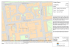### Media Kit - Hometownlife.com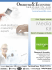### Doppler effect### PrimeFilm 1800 AFL Low-Cost 35mm Slide/Film Scanning Through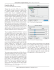### • Power and Intensity • Doppler effect for (i) mechanical waves e.g.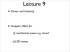### BALTICA 10